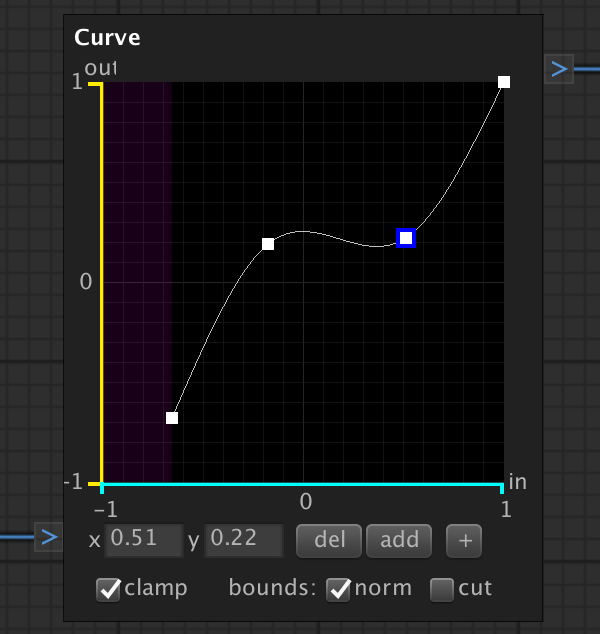# CurveNode

The CurveNode can be used to map input numbers to output numbers using a curve. Input values between -1 and 1 are are horizontally aligned and their output values are vertically aligned. Drag and drop the points of the curve to set the shape. Add and remove points using the buttons. The CurveNode is useful to modify the shape of a height map.

### Options:

 del Deletes the selected curve point. add Adds a new point at [0,0] (if this point is not already taken) + Increase the size of the node. - Decrease the size of the node. clamp Clamps the curve to [-1, 1] if checked. scale Only visible if your curves input range is lower than [-1, 1]. Scales the output range to the input range. cut Only visible if your curves input range is lower than [-1, 1]. Cuts the output range at the input range.

### Input:

 value A number between -1 and 1.

### Output:

 value The curve value at the input value.

### Example: